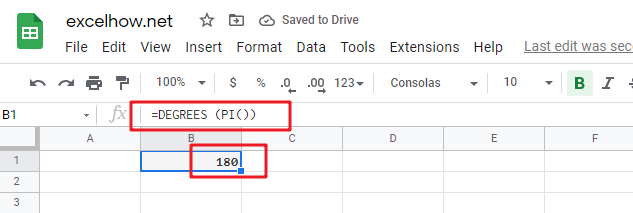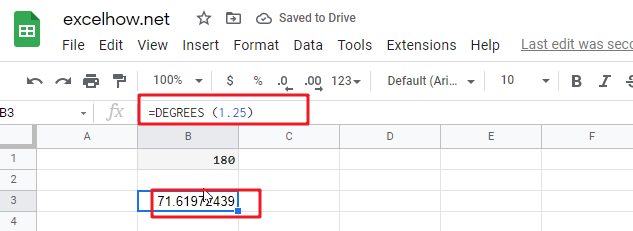This post will guide you how to use Google Sheets DEGREES function with syntax and examples.

## Description

The Google Sheets DEGREES function converts radians into degrees. And it will return a numeric value. For examples, if you pass the PI() function into the DEGREES function as its argument, and it will return 180.

The purpose of this function is used to convert radians to degrees in google sheet and it’s returned value is the degrees.

The DEGREES function is a build-in function in Google Sheets and it is categorized as a MATH function.

## Syntax

The syntax of the DEGREES function is as below:

=DEGREES (angle)

Where the DEGREES function argument is:

• angle -This is a required argument. The angle in radians that you want to convert to degrees.

## Google Sheets DEGREES Function Examples

The below examples will show you how to use google sheets DEGREES Function to convert radians into degrees.

1# get the degrees of pi radians, enter the following formula in Cell B1.

`=DEGREES (PI())`2# get the degrees of 1.25 radians, enter the following formula in Cell B2.

`=DEGREES (1.25)`Related Posts

This post will guide you how to use Google Sheets LN function with syntax and examples. Description The Google Sheets LN function returns the natural logarithm of a given number.  And the natural logarithms are based on the constant e ...

This post will guide you how to use Google Sheets INT function with syntax and examples. Description The Google Sheets INT function returns the integer portion of a given number. And it will round a given number down to the ...

This post will guide you how to use Google Sheets FLOOR function with syntax and examples. Description The Google Sheets FLOOR function returns a number rounded down to the nearest multiple of significance. So it will return a rounded number. ...

This post will guide you how to use Google Sheets EXP function with syntax and examples. Description The Google Sheets EXP function returns e raised to the power of a number.  The constant e is equal to 2.71828182845904, and it ...

This post will guide you how to use Google Sheets FACT function with syntax and examples. Description The Google Sheets FACT function returns the factorial of a given number.  The factorial of a given number is equal to 1*2*3…* number. ...

This post will guide you how to use Google Sheets EVEN function with syntax and examples. Description The Google Sheets EVEN function rounds a given number up to the nearest even integer. You can use the EVEN function to return ...

This post will guide you how to use Google Sheets COUNTBLANK function with syntax and examples. Description The Google Sheets COUNTBLANK function use to count the number of empty cells in a range of cells. You can use the COUNTBLANK ...